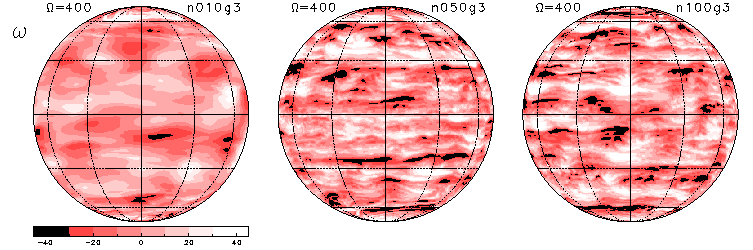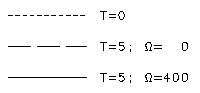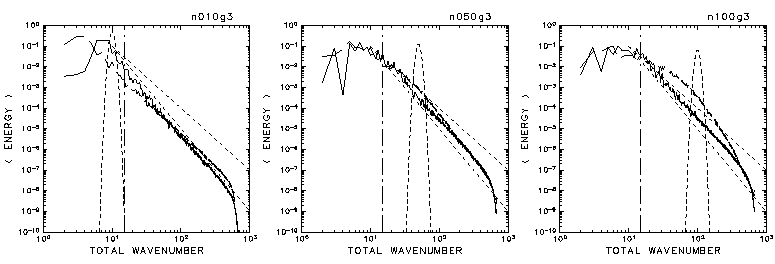3. Results

3.1 Overview of the Time Evolution of the Vorticity Field and the Zonal-Mean Zonal Flow
3.2 Generation of easterly polar vortex
3.3 The Condition Required for the Appearance of the Band Structure

### 3.3 The Condition Required for the Appearance of the Band Structure

The figures below show the final state (t = 5) of relative vorticity (ω) for 3 different initial parameters: n0 = 10, 50, and 100.

The other parameters were the same, at γ = 1000, Ω = 400, and T682.

 In the case of n0 = 10. In the case of n0 = 50. In the case of n0 = 100.• For n0 = 50 and 100, the band structure of the vorticity field elongated in the zonal direction is clearly visible. However, the band structure is not significant for n0 = 10.The figures below show the initial state (t = 0) and final state (t = 5) of the energy spectra corresponding to the respective cases above.

The additional lines denote the wavenumber of the Rhines scale (nβ: dashed-and-dotted line) and the power curves of n-3 and n-4 (dotted line).

The results for the cases of Ω = 0 for the same initial values are also shown together for the sake of reference. Refer to the legend on right to see what each of the lines denotes.

 In the case of n0 = 10. In the case of n0 = 50. In the case of n0 = 100.• In the case of n0 = 10, the peak of initial energy (n0) is smaller than the wavenumber of the Rhines scale (nβ), and no notable slope changes are observed in the structure of the energy spectrum near nβ.

• In the cases of n0 = 50 and 100, n0 is larger than nβ, and there is an observable slope change in the structure of the energy spectrum of the final state near nβ. In other words, the energy spectrum corresponds to the power curve of n ≈ - 3.5 at n ≥ nβ, but follows a more gradual power curve at n ≤ nβ.